Question

# How would you prepare the following aqueous solutions? (a) 1.42 kg of 0.0605 m ethanol (C2H5OH)...

How would you prepare the following aqueous solutions?

(a) 1.42 kg of 0.0605 m ethanol (C2H5OH) from ethanol and water

Add ___ g C2H5OH to ____ g H2O.

(b) 503 g of 10.0 mass % HCl from 30.9 mass % HCl

Add ____ g of 30.9% HCl to ____ g of water.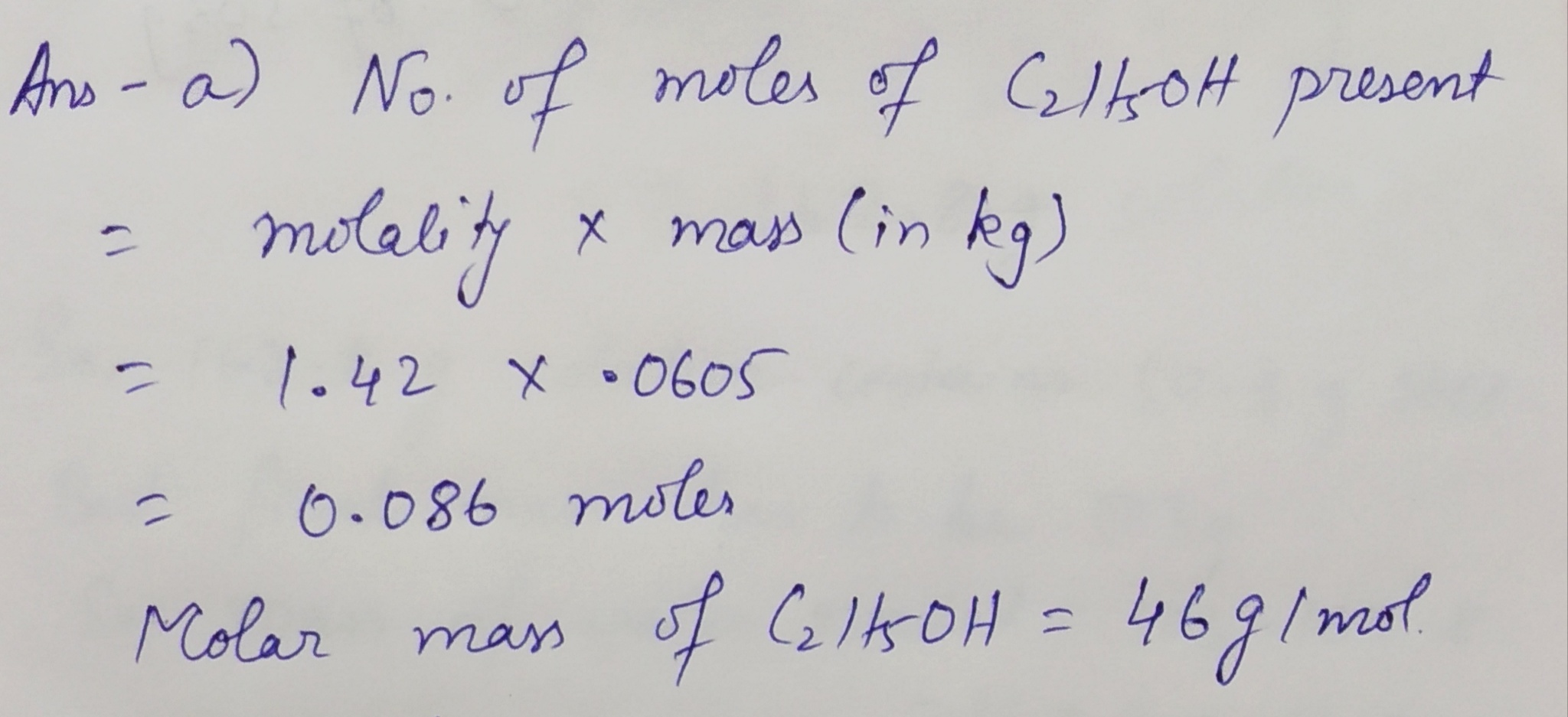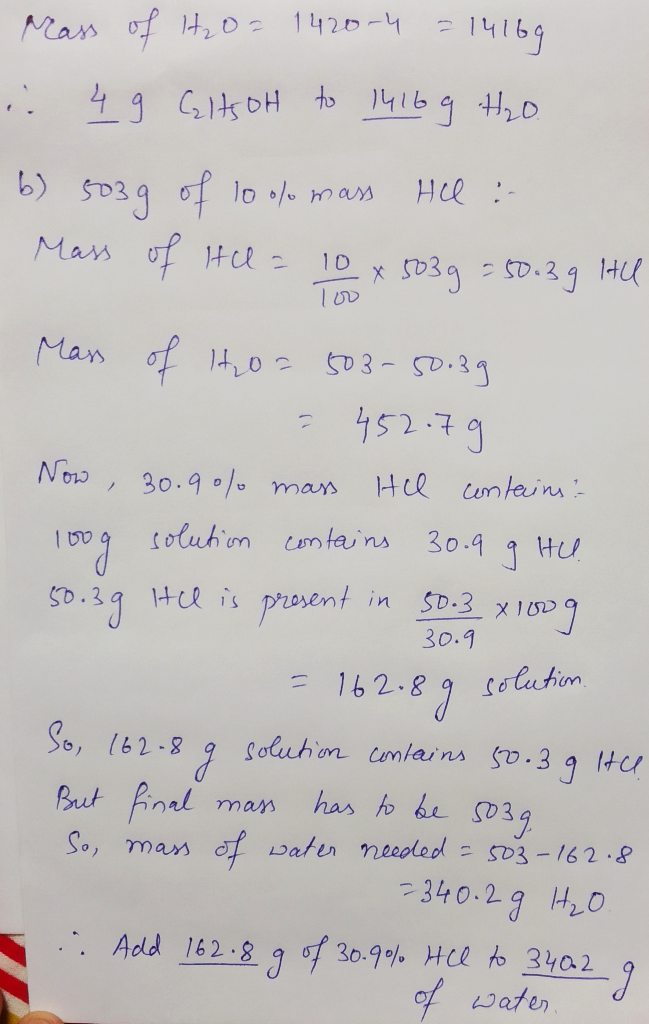#### Earn Coins

Coins can be redeemed for fabulous gifts.

Similar Homework Help Questions
• ### QUESTION 2 Data related to aqueous solutions of sodium chloride (NaCl) and aqueous solutions of ethanol...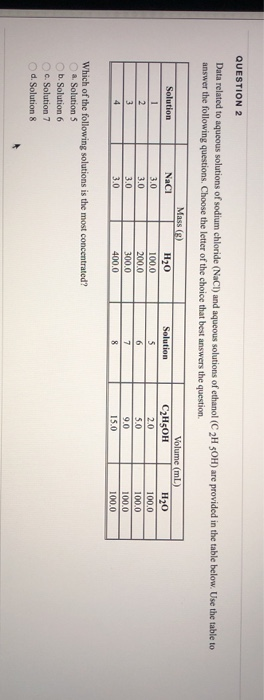QUESTION 2 Data related to aqueous solutions of sodium chloride (NaCl) and aqueous solutions of ethanol (C2H5OH) are provided in the table below. Use the table to answer the following questions. Choose the letter of the choice that best answers the question. Mass (g) Solution Solution NaCl 3.0 3.0 H20 100.0 200.0 300.0 400.0 Volume (ml) C2H5OH H20 2.0 100.0 5.0 100.0 100.0 15.0 100.0 Which of the following solutions is the most concentrated? a Solution 5 b. Solution 6...

• ### Describe how you would prepare each of the following aqueous solutions, starting with solid KBr. A....

Describe how you would prepare each of the following aqueous solutions, starting with solid KBr. A. 0.90 L of 1.4×10−2 M KBr B. 110 g of 0.160 m KBr C. 1.95 L of a solution that is 14.0 % KBr by mass (the density of the solution is 1.10 g/mL) D. a 0.130 M solution of KBr that contains just enough KBr to precipitate 16.0 g of AgBr from a solution containing 0.480 mol of AgNO3

• ### 12. Which of the following aqueous solutions would give the lowest freezing point? You must justify...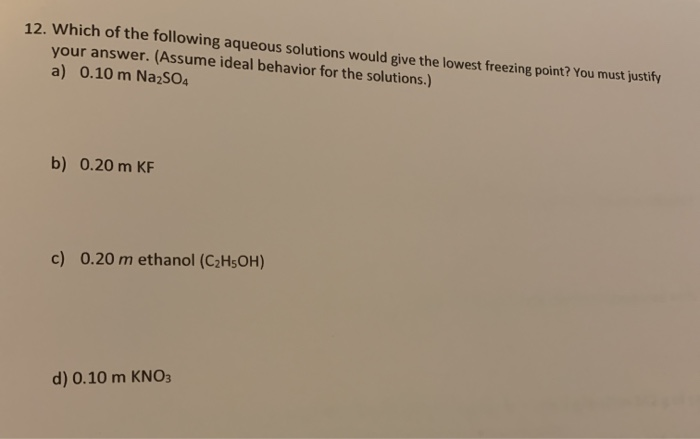12. Which of the following aqueous solutions would give the lowest freezing point? You must justify your answer. (Assume ideal behavior for the solutions.) a) 0.10 m Na2SO4 b) 0.20 m KF c) 0.20 m ethanol (C2H5OH) d) 0.10 m KNO3

• ### Calculate the molarity of each of the following solutions: (a) 33.5 g of ethanol (C2H5OH) in...

Calculate the molarity of each of the following solutions: (a) 33.5 g of ethanol (C2H5OH) in 4.30 × 102 mL of solution ?M (b) 22.6 g of sucrose (C12H22O11) in 78.5 mL of solution ?M (c) 6.90 g of sodium chloride (NaCl) in 82.4 mL of solution ?M

• ### 8. Ethanol (C2H5OH) is synthesized for industrial use by the following reaction, carried out at very...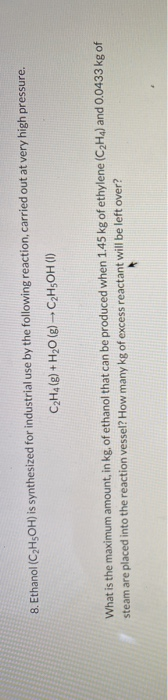8. Ethanol (C2H5OH) is synthesized for industrial use by the following reaction, carried out at very high pressure. C2H4 (g) + H2O(g) - C2H5OH (1) What is the maximum amount, in kg. of ethanol that can be produced when 1.45 kg of ethylene (C2H4) and 0.0433 kg of steam are placed into the reaction vessel? How many kg of excess reactant will be left over?

• ### Reaction Stoichiometry: Determining How Much CO2 You Produce. 1. Ethanol, C2H5OH, is a fuel additive commonly...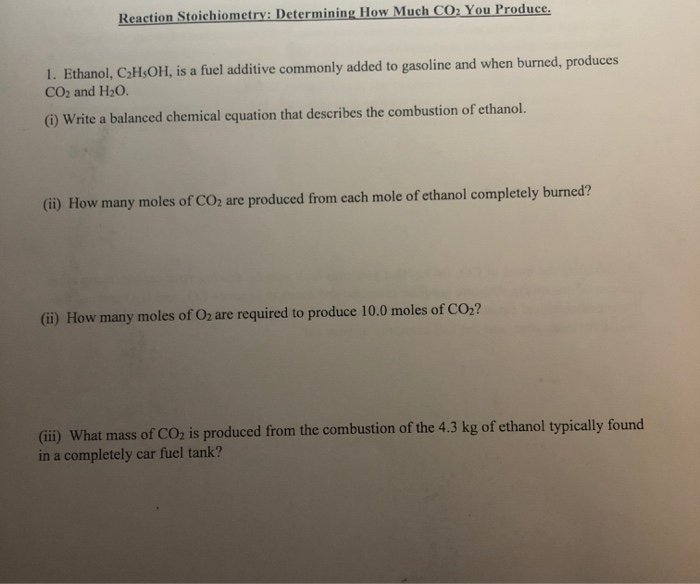Reaction Stoichiometry: Determining How Much CO2 You Produce. 1. Ethanol, C2H5OH, is a fuel additive commonly added to gasoline and when burned, produces CO2 and H20. (i) Write a balanced chemical equation that describes the combustion of ethanol. (ii) How many moles of CO2 are produced from each mole of ethanol completely burned? (ii) How many moles of O2 are required to produce 10.0 moles of CO2? (iii) What mass of CO2 is produced from the combustion of the 4.3...

• ### Describe how you would prepare each of the following aqueous solutions, starting with solid KBr. 1]...

Describe how you would prepare each of the following aqueous solutions, starting with solid KBr. 1] 0.70 L of 1.4×10−2 M KBr Express your answer using two significant figures. Enter your answers numerically separated by commas. 2] 120 g of 0.190 m KBr Enter your answers numerically separated by commas. 3] 1.70 L of a solution that is 14.0 % KBr by mass (the density of the solution is 1.10 g/mL) Enter your answers numerically separated by commas. 4] a...

• ### How much of each of the following substances would you add to water to prepare 1.10...

How much of each of the following substances would you add to water to prepare 1.10 L of a 0.20 M solution? (a) H2SO4 from "concentrated" (18 M) sulfuric acid (b) HCl from "concentrated" (12 M) reagent (c) NiCl2 from the salt NiCl2·6 H2O (d) HNO3 from "concentrated" (16 M) reagent (e) sodium carbonate from the pure solid

• ### I have 250 g of solid ethanol (C2H5OH) at -200 oC. How much energy would I...

I have 250 g of solid ethanol (C2H5OH) at -200 oC. How much energy would I need to add to get ethanol gas at 250 oC?

• ### 13.54 Describe how you would prepare each of the following aque- ous solutions: (a) 1.50 L...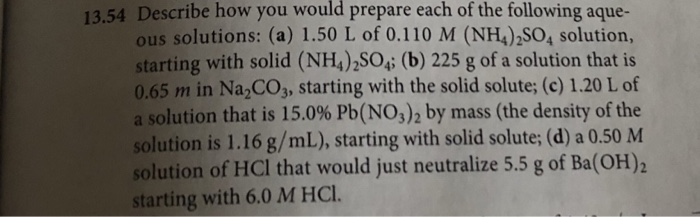13.54 Describe how you would prepare each of the following aque- ous solutions: (a) 1.50 L of 0.110 M (NH4)2SO4 solution, starting with solid (NH4)2SO4; (b) 225 g of a solution that is 0.65 m in Na2CO3, starting with the solid solute; (c) 1.20 L of a solution that is 15.0% Pb(NO3) 2 by mass (the density of the solution is 1.16 g/mL), starting with solid solute; (d) a 0.50 M solution of HCI that would just neutralize 5.5 g...